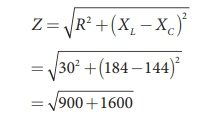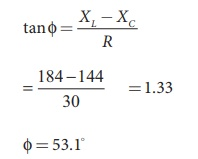Home | | Physics 12th Std | Solved Example Problems on Alternating Current (AC) and Circuit

# Solved Example Problems on Alternating Current (AC) and Circuit

Physics : Alternating Current: Alternating Current (AC) and Circuit: Solved Example Problems with Answers, Solution and Explanation

## RMS value of AC: Solved Example Problems

EXAMPLE 4.18

Write down the equation for a sinusoidal voltage of 50 Hz and its peak value is 20 V. Draw the corresponding voltage versus time graph.

SolutionEXAMPLE 4.19

The equation for an alternating current is given by i = 77 sin 314t. Find the peak value, frequency, time period and instantaneous value at t = 2 ms.

Solution

i = 77 sin 314t ; t = 2 ms = 2├Ś10-3 s

The general equation of an alternating current is i = Im sin Žēt . On comparsion,

(i) Peak value, Im = 77 A

(ii) Frequency, f = Žē/2ŽĆ = 314 / 2 ├Ś3.14 = 50 Hz

Time period, T = 1/f = 150 = 0 .02 s

(iv) At t = 2 m s,

Instantaneous value,

i = 77sin(314├Ś2├Ś10ŌłÆ3 )

i = 45.24 A

## AC circuit containing only an inductor: Solved Example Problems

EXAMPLE 4.20

A 400 mH coil of negligible resistance is connected to an AC circuit in which an effective current of 6 mA is flowing. Find out the voltage across the coil if the frequency is 1000 Hz.

Solution

L = 400 x 10-3 H; Ieff = 6 x 10-3A

f = 1000 Hz## AC circuit containing only a capacitor: Solved Example Problems

EXAMPLE 4.21

A capacitor of capacitance 102/ŽĆ ┬ĄF is connected across a 220 V, 50 Hz A.C. mains. Calculate the capacitive reactance, RMS value of current and write down the equations of voltage and current.

Solution## Quality factor or QŌĆōfactor: Solved Example Problems

EXAMPLE 4.22

Find the impedance of a series RLC circuit if the inductive reactance, capacitive reactance and resistance are 184 ╬®, 144 ╬® and 30 ╬® respectively. Also calculate the phase angle between voltage and current.

Solution

XL = 184 ╬®; XC = 144 ╬®

R = 30 ╬®

(i ) The impedance isImpedance, Z = 50 Ōä”

(ii) Phase angle isŽå = 53.1

EXAMPLE 4.23

A 500 ╬╝H inductor, 80/ŽĆ2 pF capacitor and a 628 ╬® resistor are connected to form a series RLC circuit. Calculate the resonant frequency and Q-factor of this circuit at resonance.

Solution

L=500├Ś10-6H; C = 80/ŽĆ2 ├Ś10ŌłÆ12 F; R = 628Ōä”

(i) Resonant frequency isQ =12.5

EXAMPLE 4.24

Find the instantaneous value of alternating voltage Žģ = 10 sin(3 ŽĆ├Ś104 t) volt at i) 0ŌĆås ii) 50 ╬╝s iii) 75 ╬╝s.

Solution

The given equation is Žģ = 10sin (3 ŽĆ├Ś104 t)EXAMPLE 4.25

The current in an inductive circuit is given by 0.3 sin (200t ŌĆō 40┬░) A. Write the equation for the voltage across it if the inductance is 40 mH.

Solution

L = 40 ├Ś 10-3 H; i = 0.1 sin (200t ŌĆō 40┬║)

XL = ŽēL = 200 ├Ś 40 ├Ś 10-3 = 8 Ōä”

Vm = Im XL = 0.3 ├Ś 8 = 2.4 V

In an inductive circuit, the voltage leads the current by 90o Therefore,

v = Vm sin ( Žēt +90┬║)

v = 2 . 4 sin(200t ŌłÆ40 + 90 ┬║)

v = 2 . 4 sin(200t +50 ┬║)volt

Study Material, Lecturing Notes, Assignment, Reference, Wiki description explanation, brief detail
12th Physics : Electromagnetic Induction and Alternating Current : Solved Example Problems on Alternating Current (AC) and Circuit |